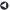### Statistical Services OfferedBack to List of Services

#### Categorical Data Analysis including Predictive Modeling

Categorical Data Analysis deals with categorical outcomes, regardless of whether the predictor variables are categorical or continuous. Continuous Data Analysis deals with continuous outcomes, regardless of whether the predictor variables are categorical or continuous.

When the outcome has two categories (or levels or values) such as "Yes" or "No," the response variable is binary or dichotomous. When the outcome has three or more categories, the response variable can be nominal or ordinal. Nominal variables have values with no logical ordering. Ordinal variables have values with a logical order; however, the relative distances between the values are not clear. Continuous variables, in contrast, have values with a logical order, and the relative distances between the values are meaningful.

Regression analysis is used to characterize the relationship between the response variable and one or more predictor variables. In linear regression, the response variable is continuous. In logistic regression, the response variable is categorical. Logistic regression analysis uses the predictor variables, which can be categorical or continuous, to predict specific outcomes of the response variable. That is, logistic regression gives probabilities of the values of the response variable.

When the response variable is categorical, linear regression produces invalid results for two major reasons:

1. The predicted values from a linear model rarely fall only between 0 and 1. However, probabilities by definition have values from 0 to 1. A more suitable model than the linear model would constrain the predicted probabilities to be between 0 and 1.
2. The observed relationship between the outcome and the predictor variables is usually nonlinear rather than linear, often resembling an S-shaped curve.

To deal with the nonlinear relationship between the probabilities of the outcomes and the predictor variables, the logistic model applies the logit transformation to the probabilities. The logit is the log of the odds. (The odds is the ratio of the probability of the outcome to the probability of no outcome.) The logit transformation results in a linear relationship with the predictor variables and ensures the model gives estimated probabilities between 0 and 1.

When effect coding (also called deviation from the mean coding) is used for the Class variables, parameter estimates of the Class main effects estimate the difference between each level (or value) of an effect and the average of that effect over all levels. In reference cell coding, parameter estimates of the Class main effects estimate the difference between each level of an effect and the assigned reference level of that effect.

The SAS Logistic procedure can handle a range of logistic regression analysis, including binary, ordinal, and nominal responses (or outcomes). In binary logistic regression, one intercept and one set of parameters are estimated for one logit function.

Ordinal logistic regression (i.e., when the response variable has three or more ranked categorical levels) is handled in Proc Logistic by the proportional odds model which calculates cumulative logits. They yield cumulative probabilities, which is the probability that a subject is in an indicated category or lower. A separate intercept is estimated for each cumulative logit. However, a separate slope is not estimated for each cumulative logit, but rather a common slope is estimated across the cumulative logits for each predictor variable. This common slope is a weighted average across the logits. A parallel-lines-regression model is fitted, with each curve (which describes the cumulative probabilities) having the same shape. The only difference in the curves is the difference between the values of the intercept parameters.

Nominal logistic regression (i.e., when the response variable has categorical values with no logical ordering) is handled in Proc Logistic by generalized logits. Multiple sets of parameters are estimated for both the intercept and explanatory variables.

Repeated Measures Data Analysis: When the response variable is categorical and repeated measurements are taken on a subject, special statistical methods are required because the set of measurements on one subject may be correlated. Correlation must be taken into account to draw valid inferences. Analyses using repeated measurements of categorical response variables are part of longitudinal data analysis and are handled in SAS using Proc GenMod. (See section below entitled "Longitudinal Data Analysis.")

Predictive Modeling is an application of logistic regression in which the response variable is usually binary and the overriding goal is prediction of group membership rather than statistical inference. There are many business uses of predictive modeling including target marketing, attrition prediction, credit scoring, and fraud detection.

Predictive modeling uses observations (or cases) for which both the values of the predictor variables and the value of the target (or outcome or response) variable are known to develop a model for predicting the target variable for cases when only values of the predictor variables are known. Such predicting is commonly referred to as "scoring new cases." The result of the process is a (posterior) probability for each case of whether it belongs to class 0 or class 1. An allocation rule is then developed with a cutoff probability where cases above the cutoff are assigned to class 1 and cases below the cutoff are assigned to class 0.

A common problem in predictive modeling is large numbers (hundreds) of predictor variables, including categorical variables with many levels. Proc Varclus can used to eliminate redundant numeric predictor variables. Proc Cluster can be used for collapsing the levels of categorical predictor variables.Back to List of Services